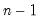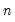scores
Compute factor score coefficients and scores and display results in table, sheet, or graph form.
Syntax
There are two forms of the scores command. The first form of the command, which applies when displaying table results or spreadsheet displays of scores is given by:
factor_name.scores(options) [observed_list]
The optional observed_list of observed input variables will be multiplied by the score coefficients to compute the scores.
The second form of the command applies when plotting scores. In this case, the syntax is:
factor_name.scores(options) [graph_list] [@ observed_list]
where the [graph_list] is an optional list of integers and/or vectors containing integers identifying the factors to plot. If graph_list is not provided, EViews will construct graphs using all of the retained factors.
Multiple pairs are handled using the method specified in the “mult=” option. Note that the order of elements in the list matters; reversing the order of two indices reverses the axis on which each factor is displayed.
You should also bear in mind that:
Specification of the observed_list is required only for actually computing score values—it is not required for computing score coefficient summaries and diagnostics (“out=table”).
If observed_list is not provided, EViews will use the observed variables from the factor estimation specification. For factor models specified using a symmetric matrix, you must provide a observed_list if you wish to obtain score values.
Scores values will be computed for observations in the current workfile sample that do not have missing values for the observed inputs.
Options

 out=arg (default=“table”) Output format: coefficient summary and diagnostics (“table”), spreadsheet table of scores (“sheet”), graph of scores (“graph”), graph of scores with loadings axes (“biplot”). unrotated Use unrotated loadings in computations (the default is to use the rotated loadings, if available). type =arg (default=“exact”) Exact coefficient (“exact”), coarse adjusted factor coefficients (“coefs”), coarse adjusted factor loadings (“loadings”). coef=arg (default=“reg”) Method for computing the exact or coarse adjusted factor score coefficient matrix: Thurstone regression (“reg”), Ideal Variables (“ideal”), Bartlett weighted least squares (“wls”), generalized Anderson-Rubin-McDonald (“anderson”), Green (“green”).For “type=exact” and “type=coefs” specifications. coarse=arg (default=“unrestrict”) Method for computing the coarse (-1, 0, 1) scores coefficients (Grice, 1991a):Unrestricted -- (“unrestrict”) coef weights set based only on sign; Unique–recode (“recode”) only element with highest value is coded to a non-zero value; Unique–drop (“drop”) only elements with loadings not in excess of the threshold are set to non-zero values.For “type=coefs” and “type=loadings” specifications. cutoff=number (default = 0.3) Cutoff value for coarse scores coefficient calculations (Grice, 1991a). For “type=coefs” specifications, the cutoff value represents the fraction of the largest absolute coefficient weight per factor against which the exact score coefficients should be compared.For “type=loadings” specifications, the cutoff is the value against which the absolute loadings or structure coefficients should be compared. moment=arg (default =“est”; if feasible) Standardize the observables data using means and variances from: original estimation (“est”), the computed moments from specified observable variables (“obs”).The “moment=est” option is only available for factor models estimated using Pearson or uncentered Pearson correlation and covariances since the remaining models involve unobserved or non-comparable moments. df Degrees-of-freedom correct the observables variances computed when “moment=obs” (divide sums-of-squares byinstead of). coefout (Optional) Name of matrix in which to save factor score coefficient matrix. prompt Force the dialog to appear from within a program. p Print results.
Graph Options

 mult =arg (default=“first”) Multiple series handling for graphs: plot first against remainder (“first”), plot as x-y pairs (“pair”), lower-triangular plot (“lt”) nocenter Do not center graphs around the origin. labels=arg, (default=“outlier”) Observation labels for scores: outliers only (“outlier”), all points (“all”), none (“none”). labelprob=number Probability value for determining whether a point is an outlier according to the chi-square tests based on the squared Mahalanbois distance between the observation and the sample means (when using the “labels=outlier” option). userscale=arg User-scale factor to be applied to the unscaled loadings (setting this option overrides the automatic scaling). autoscale=arg (default = 1) User-scale factor to be applied to the automatic loadings scale (when displaying both loadings and scores).
Examples
f1.scores(out=table)
computes factor score coefficients and displays a table of coefficient summaries and diagnostics.
f1.scores(coef=anderson, out=biplot, mult=first) 1 3 4
displays a biplot graph of the factor scores. The graph plots the first factor against the third, and the first factor against the fourth. The scores are computed using the observed variables from the original factor estimation specification and generalized Anderson-Rubin-McDonald factor score coefficients.
Cross-references
See “Estimating Scores” and “Scoring”.